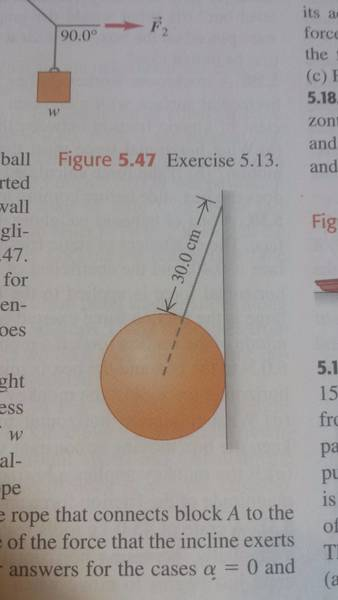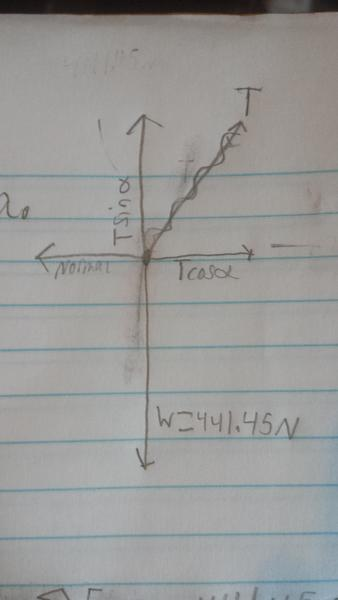# Tension of wire attached to 45-kg ball.

• BlueQuark

## Homework Statement

"A solid uniform 45-kg ball of diameter 32cm is supported against a verticle frictionless wall using a thin 30cm wire of negligible mass.

A)Make a free body diagram for the ball and use it to find the tension in the wire.
B)How hard does the ball push against the wall?[/B]

## Homework Equations

##\sum(Fx) = 0##
##\sum(Fy) = 0##
##a^2 + b^2 = c^2##
[/B]

## The Attempt at a SolutionThere is a picture of the situation.

Here is another picture of my free body diagram.Now, using the equilibrium equations, I got ##\sum(Fy) = -441.45 + TSinθ = 0##
So, ##Tsinθ = 441.45## (Newtons).

Now for the x components.
##\sum(Fx) = Tcosθ - N = 0##

So, ##Tcosθ = N##

Now I need to figure out what the angle is. The diameter of the sphere is 32cm, so the radius must be 16cm. The length of the wire is 30cm. I used the pythagorean theorem to find the height from the sphere to the wire.

##16^2 + b^2 = 30^2##
##256 + b^2 = 900##
##b^2 = 644##
##b = 25.38##

Now ##\arcsin(25.38/30) = 57.78 degrees##

This is where I start to have trouble. I figured I would find T by plugging that angle into ##Tsinθ = 441.45## and solving for T. This gave me an answer of 521.8 N. When I check the back of the book, the answer is actually 470 Newtons.

Any help?

## Homework Statement

"A solid uniform 45-kg ball of diameter 32cm is supported against a verticle frictionless wall using a thin 30cm wire of negligible mass.

A)Make a free body diagram for the ball and use it to find the tension in the wire.
B)How hard does the ball push against the wall?[/B]

## Homework Equations

##\sum(Fx) = 0##
##\sum(Fy) = 0##
##a^2 + b^2 = c^2##
[/B]

## The Attempt at a SolutionThere is a picture of the situation.

Here is another picture of my free body diagram.Now, using the equilibrium equations, I got ##\sum(Fy) = -441.45 + TSinθ = 0##
So, ##Tsinθ = 441.45## (Newtons).

Now for the x components.
##\sum(Fx) = Tcosθ - N = 0##

So, ##Tcosθ = N##

So far, so good.
Now I need to figure out what the angle is. The diameter of the sphere is 32cm, so the radius must be 16cm. The length of the wire is 30cm. I used the pythagorean theorem to find the height from the sphere to the wire.

##16^2 + b^2 = 30^2##
##256 + b^2 = 900##
##b^2 = 644##
##b = 25.38##

Now ##\arcsin(25.38/30) = 57.78 degrees##

This is where everything comes apart.

You should make a detailed sketch of the triangle you are trying to solve. Even looking at the picture from the book should give you a hint that your first equation,
##16^2 + b^2 = 30^2##
is wrong.

Take another close look at the geometry of the sphere and see if you can't come up with a different (and correct) relationship.

So far, so good.

This is where everything comes apart.

You should make a detailed sketch of the triangle you are trying to solve. Even looking at the picture from the book should give you a hint that your first equation,
##16^2 + b^2 = 30^2##
is wrong.

Take another close look at the geometry of the sphere and see if you can't come up with a different (and correct) relationship.

Okay, I looked and found the mistake. The 30 cm hypotenuse only went from the top to the center of the ball. I should have added an extra 16 cm to it to go through the entire sphere. I got the right answer. Thank you for your help!